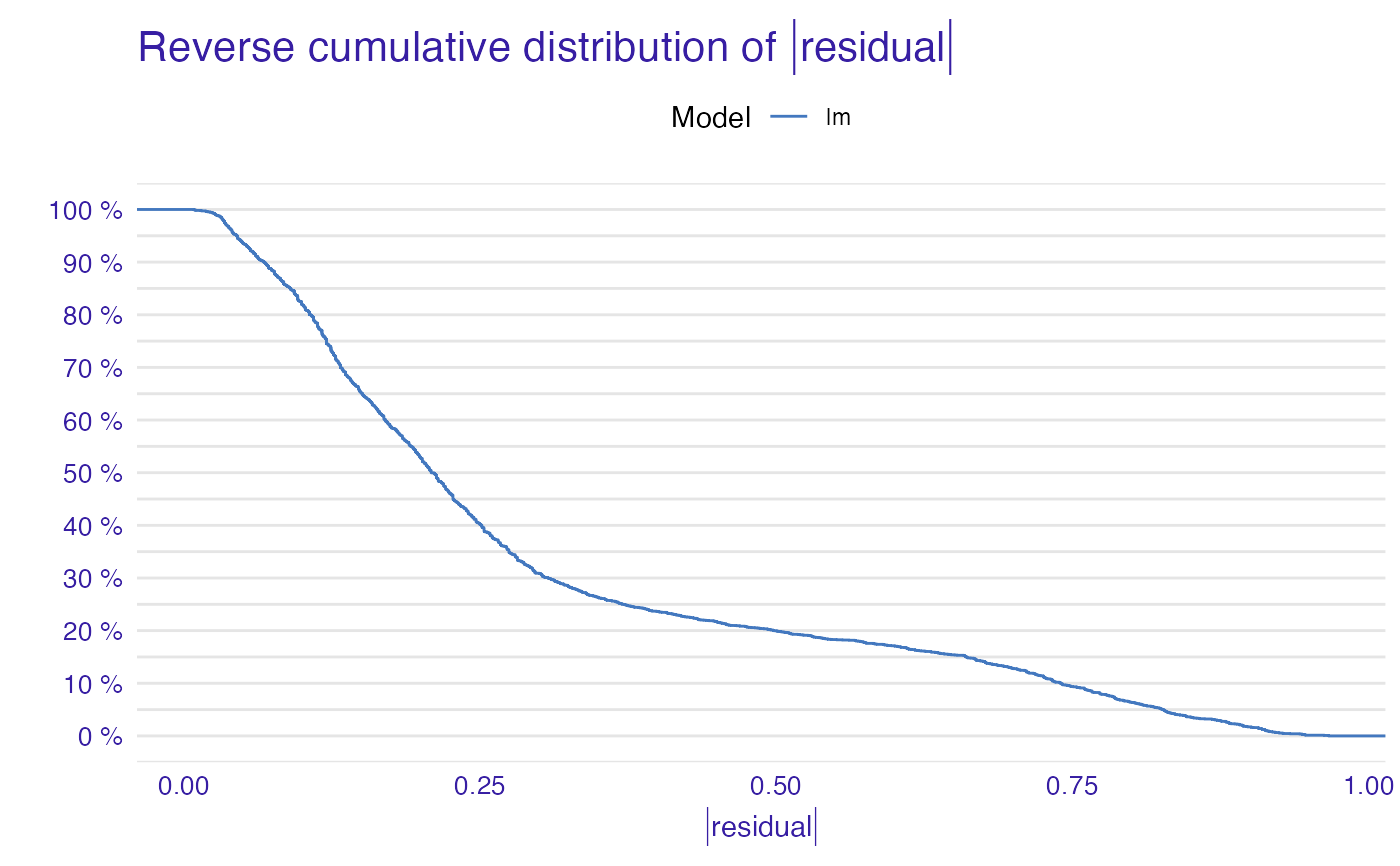Creates auditor_model_performance object that can be used to plot radar with ranking of models.

model_performance(
object,
score = c("mae", "mse", "rec", "rroc"),
new_score = NULL,
data = NULL,
...
)

modelPerformance(
object,
score = c("mae", "mse", "rec", "rroc"),
new_score = NULL
)

## Arguments

object An object of class explainer created with function explain from the DALEX package. Vector of score names to be calculated. Possible values: acc, auc, cookdistance, dw, f1, gini, halfnormal, mae, mse, peak, precision, r2, rec, recall, rmse, rroc, runs, specificity, one_minus_acc, one_minus_auc, one_minus_f1, one_minus_gini, one_minus_precision, one_minus_recall, one_minus_specificity (for detailed description see functions in see also section). Pass NULL if you want to use only custom scores by new_score parameter. A named list of functions that take one argument: object of class 'explainer' and return a numeric value. The measure calculated by the function should have the property that lower score value indicates better model. New data that will be used to calculate scores. Pass NULL if you want to use data from object. Other arguments dependent on the score list.

## Value

An object of the class auditor_model_performance.

score_acc, score_auc, score_cooksdistance, score_dw, score_f1, score_gini, score_halfnormal, score_mae, score_mse, score_peak, score_precision, score_r2, score_rec, score_recall, score_rmse, score_rroc, score_runs, score_specificity, score_one_minus_acc, score_one_minus_auc, score_one_minus_f1, score_one_minus_precision, score_one_minus_gini, score_one_minus_recall, score_one_minus_specificity

## Examples

data(titanic_imputed, package = "DALEX")

# fit a model
model_glm <- glm(survived ~ ., family = binomial, data = titanic_imputed)

# use DALEX package to wrap up a model into explainer
glm_audit <- audit(model_glm,
data = titanic_imputed,
y = titanic_imputed\$survived)
#> Preparation of a new explainer is initiated
#>   -> model label       :  lm  (  default  )
#>   -> data              :  2207  rows  8  cols
#>   -> target variable   :  2207  values
#>   -> predict function  :  yhat.glm  will be used (  default  )
#>   -> predicted values  :  No value for predict function target column. (  default  )
#>   -> model_info        :  package stats , ver. 4.1.1 , task classification (  default  )
#>   -> predicted values  :  numerical, min =  0.008128381 , mean =  0.3221568 , max =  0.9731431
#>   -> residual function :  difference between y and yhat (  default  )
#>   -> residuals         :  numerical, min =  -0.9628583 , mean =  -2.569729e-10 , max =  0.9663346
#>   A new explainer has been created!
# validate a model with auditor
library(auditor)
mp <- model_performance(glm_audit)
mp
#> Measures for:  classification
#> recall     : 0.5738397
#> precision  : 0.7472527
#> f1         : 0.6491647
#> accuracy   : 0.8001812
#> auc        : 0.8115462
#>
#> Residuals:
#>          0%         10%         20%         30%         40%         50%
#> -0.96285832 -0.32240247 -0.23986439 -0.19544185 -0.14842925 -0.11460334
#>         60%         70%         80%         90%        100%
#> -0.06940964  0.06185475  0.29607060  0.72120412  0.96633458
plot(mp)